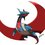# New equation of prime-zeta? part 1

consider the arithmetic function

$Aa(n)=\begin{cases} 1 &, \text{n is prime}\\ 0&,\text{otherwise}\end{cases}$

Read dirichlet convolution and dirichlet series . we have $ya=\mu*Aa=\sum_{p|n} \mu\left(\dfrac{n}{p}\right)$ This is because $Aa$ disappears over non-primes. Consider the case

1. n is square free.then $\mu\left(\dfrac{n}{p}\right)=-\mu(n)$ and when we add over all prime factors we get $-\omega(n)\mu(n)$.

2. Not squarefree but only one prime factor is squared. then it's möbius will be zero everywhere except the repeated prime factor. so we get $(-1)^{\omega(n)}$.

3. more than one prime factor is squared. then it will simply be zero.

so: $ya(n)=\begin{cases} -k(-1)^k&, n=p_1p_2p_3...p_k\\ (-1)^k &,n=p_1p_2p_3...p_{k-1}p_k^2\\ 0 &,\text{otherwise}\end{cases}$ I will continue this in part 2. Reshare if you enjoyed this.

btw $\omega(n)$ is the nmber of prime factors of n and $\mu(n)$ is the möbius function

part-2Note by Aareyan Manzoor
5 years, 5 months ago

This discussion board is a place to discuss our Daily Challenges and the math and science related to those challenges. Explanations are more than just a solution — they should explain the steps and thinking strategies that you used to obtain the solution. Comments should further the discussion of math and science.

When posting on Brilliant:

• Use the emojis to react to an explanation, whether you're congratulating a job well done , or just really confused .
• Ask specific questions about the challenge or the steps in somebody's explanation. Well-posed questions can add a lot to the discussion, but posting "I don't understand!" doesn't help anyone.
• Try to contribute something new to the discussion, whether it is an extension, generalization or other idea related to the challenge.
• Stay on topic — we're all here to learn more about math and science, not to hear about your favorite get-rich-quick scheme or current world events.

MarkdownAppears as
*italics* or _italics_ italics
**bold** or __bold__ bold
- bulleted- list
• bulleted
• list
1. numbered2. list
1. numbered
2. list
Note: you must add a full line of space before and after lists for them to show up correctly
paragraph 1paragraph 2

paragraph 1

paragraph 2

[example link](https://brilliant.org)example link
> This is a quote
This is a quote
    # I indented these lines
# 4 spaces, and now they show
# up as a code block.

print "hello world"
# I indented these lines
# 4 spaces, and now they show
# up as a code block.

print "hello world"
MathAppears as
Remember to wrap math in $$ ... $$ or $ ... $ to ensure proper formatting.
2 \times 3 $2 \times 3$
2^{34} $2^{34}$
a_{i-1} $a_{i-1}$
\frac{2}{3} $\frac{2}{3}$
\sqrt{2} $\sqrt{2}$
\sum_{i=1}^3 $\sum_{i=1}^3$
\sin \theta $\sin \theta$
\boxed{123} $\boxed{123}$

## Comments

Sort by:

Top Newest

I am writing part two now, where i will show the connection to prime zeta.

- 5 years, 5 months ago

Log in to reply

×

Problem Loading...

Note Loading...

Set Loading...